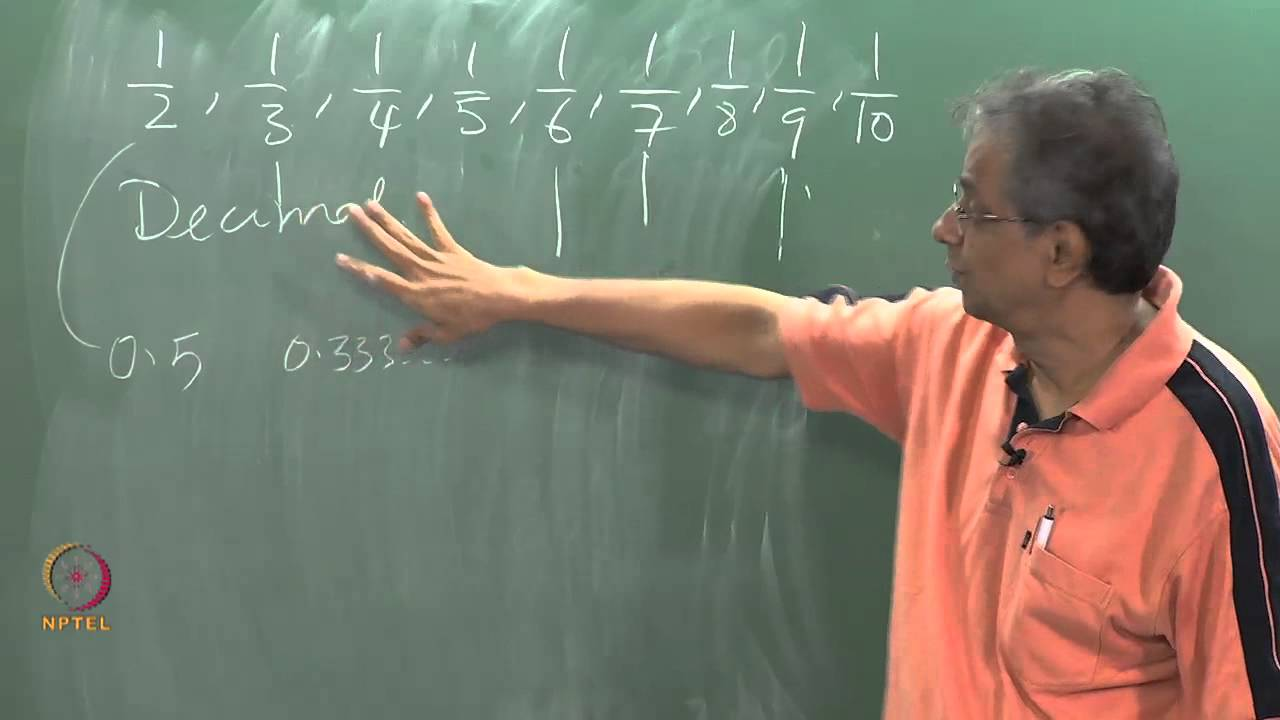NPTEL Courses

# Introduction to Computational Fluid Dynamics

Representation of mathematical ideas on the computer: numbers, functions, derivative, differential equations. Simple Problems: Solution to Laplace equation, one-dimensional first order wave equation, heat equation, finite difference schemes - stability and consistency, dissipation dispersion, finite volume method. One-dimensional Euler equations: Discretisation, Delta form, application of boundary conditions. Advanced topics: Roe's averaging, Multigrid Methods, SOR and variational techniques. (from nptel.ac.in )

Instructor

###### Prof. M. Ramakrishna, Department of Aerospace Engineering, IIT Madras.
Aeronautics and astronautics
2015

#### Lecture 01 - Introduction, Why and How We Need Computers